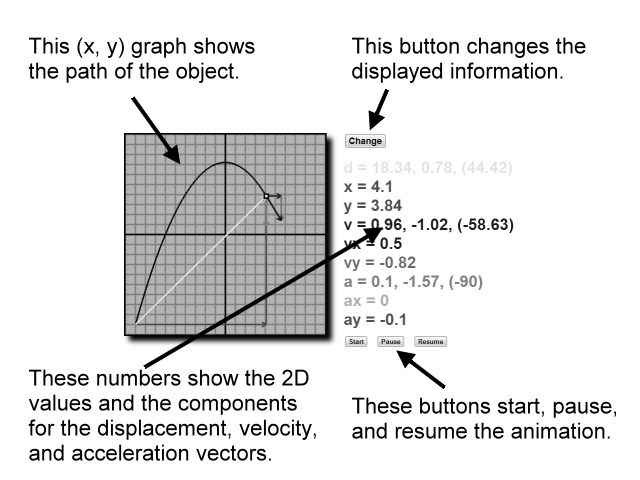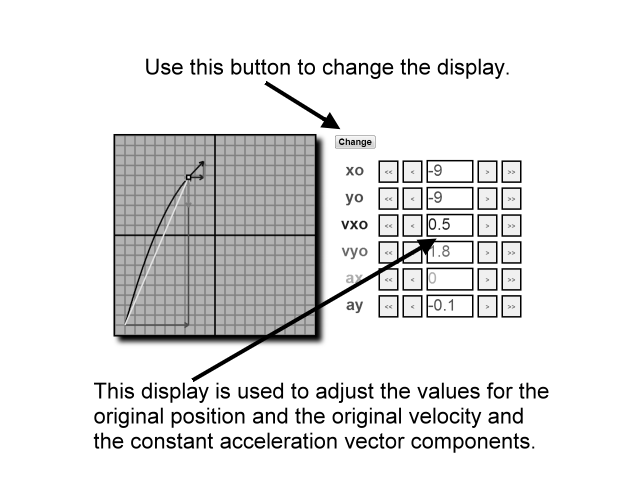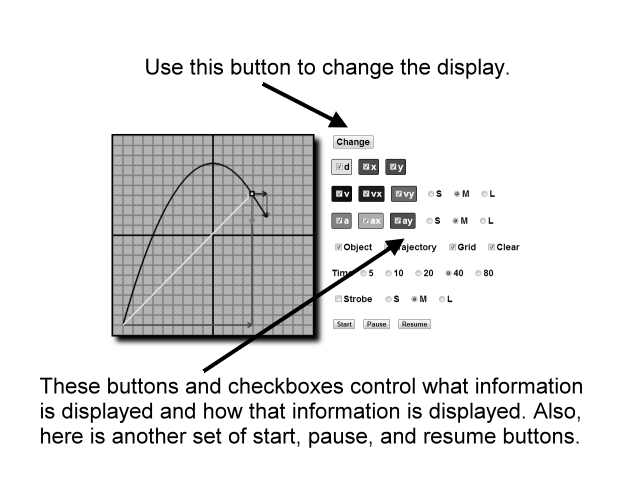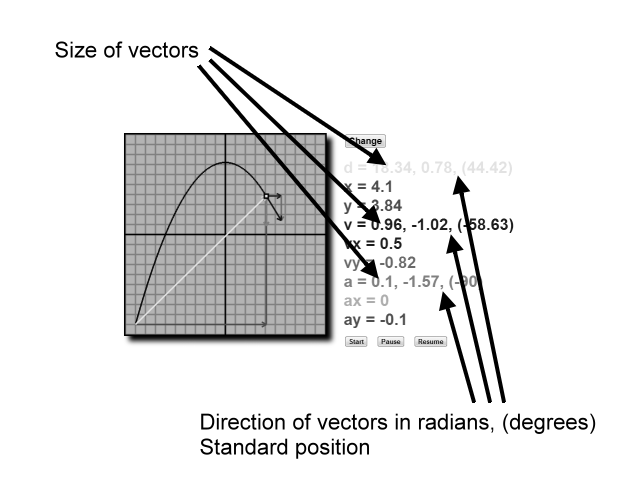# 2D Motion Looper

This is a two dimensional constant acceleration motion demonstrator - The 2D Motion Looper.

d = 0, 0
x = 0
y = 0
v = 0, 0
vx = 0
vy = 0
a = 0, 0
ax = 0
ay = 0

Time

This program shows the path, or trajectory, of an object that is accelerating. It also shows the position, velocity, and acceleration vectors for the motion.

The motion of the object recycles again and again, thus the name 'Looper'. You can change the initial parameters for the motion and then see how these changes effect the path of the object. Working with several examples can help you understand the relationships between position, velocity, and acceleration.

Quick start, first panel:We have an (x, y) position graph on the left. On the right there are actually three panels to look at, but you only see one of them at a time. The 'Change' button cycles through the right panels.

At first the right panel displays the values for the position, velocity, and acceleration vectors of the motion.

Second panel:Click the 'Change' button and go to the next right panel. With this panel you can change the original values for the x- and y-components of the position, velocity, and acceleration.

Third panel:Click 'Change' again and you will see many checkboxes and buttons. These all control what vectors are shown and how they are shown.

Here is a guide to the symbols used in this program:

• xo - The original x-position
• yo - The original y-position
• x - The current x-position
• y - The current y-position
• d - The current two dimensional displacement vector
• vxo - The original velocity in the x-direction
• vyo - The original velocity in the y-direction
• vx - The current x-component for the velocity, the x-velocity
• vy - The current y-component for the velocity, the y-velocity
• v - The current two dimensional velocity vector
• ax - The constant acceleration in the x-direction, the x-acceleration
• ay - The constant acceleration in the y-direction, the y-direction
• a - The constant two dimensional acceleration vector

The values for the two dimensional vectors are displayed with their size and direction. The size is first, followed by the direction as a radian angle in standard position, followed by the direction in degrees in standard position within parentheses:Custom Search